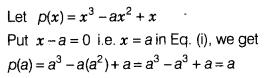# Find the remainder, when p(x) =x3- ax2 + x is divided by (x - a)

Find the remainder, when p(x) =x3- ax2 + x is divided by (x - a).